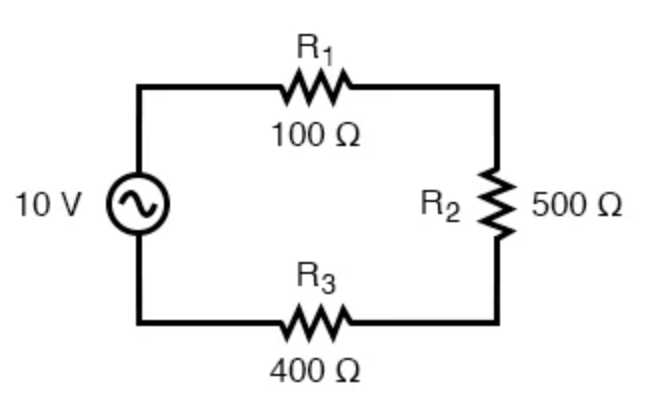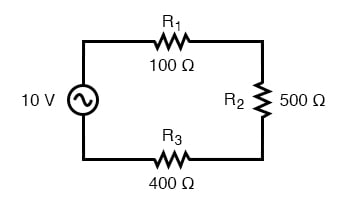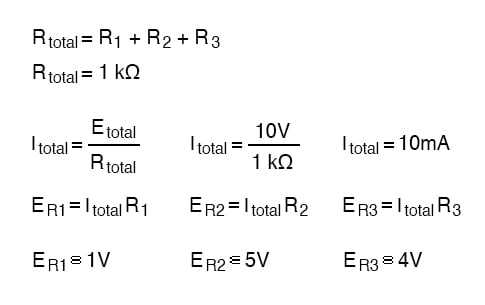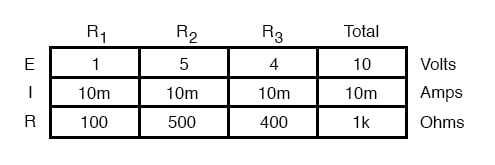## Does Ohm’s Law, Kirchhoff’s Voltage and Current Laws apply to AC?

Yes. but, the AC quantities may need to be represented in more complex form.

# Simple AC Circuit Calculations

## Chapter 1 - Basic AC Theory

Over the course of the next few chapters, you will learn that AC circuit measurements and calculations can get very complicated due to the complex nature of alternating current in circuits with inductance and capacitance.

However, with simple circuits (figure below) involving nothing more than an AC power source and resistance, the same laws and rules of DC apply simply and directly.AC circuit calculations for resistive circuits are the same as for DC.Series resistances still add, parallel resistances still diminish, and the Laws of Kirchhoff and Ohm still hold true. Actually, as we will discover later on, these rules and laws always hold true, it’s just that we have to express the quantities of voltage, current, and opposition to current in more advanced mathematical forms.

With purely resistive circuits, however, these complexities of AC are of no practical consequence, and so we can treat the numbers as though we were dealing with simple DC quantities.

Because all these mathematical relationships still hold true, we can make use of our familiar “table” method of organizing circuit values just as with DC:One major caveat needs to be given here: all measurements of AC voltage and current must be expressed in the same terms (peak, peak-to-peak, average, or RMS). If the source voltage is given in peak AC volts, then all currents and voltages subsequently calculated are cast in terms of peak units.

If the source voltage is given in AC RMS volts, then all calculated currents and voltages are cast in AC RMS units as well. This holds true for any calculation based on Ohm’s Laws, Kirchhoff’s Laws, etc. Unless otherwise stated, all values of voltage and current in AC circuits are generally assumed to be RMS rather than peak, average, or peak-to-peak.

In some areas of electronics, peak measurements are assumed, but in most applications (especially industrial electronics) the assumption is RMS.

REVIEW:

• All the old rules and laws of DC (Kirchhoff’s Voltage and Current Laws, Ohm’s Law) still hold true for AC. However, with more complex circuits, we may need to represent the AC quantities in more complex form. More on this later, I promise!
• The “table” method of organizing circuit values is still a valid analysis tool for AC circuits.

RELATED WORKSHEETS:

• Share
Published under the terms and conditions of the Design Science License